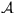Next: The Draw-a-Secret (DAS) Scheme Up: The Design and Analysis Previous: Introduction

# Textual Passwords with Graphical Assistance

In this section we present a password selection and input scheme which uses textual passwords augmented by some minimal graphical capabilities that enable the decoupling of temporal order of input and the position in which characters are input. This scheme is interesting because it simply demonstrates the power of graphical input abilities while yielding a scheme that is convincingly stronger than textual passwords are today.

We start by defining a normal, k-character textual password as a total function, whereis the set of allowed characters for the textual password. Intuitively, the domain ofdenotes the temporal order of inputs, so that the user first enters, then, and so on. That is, for a password tomato'', we have,,,,, and.

Now suppose that the user is presented with a simple graphical input display consisting of, say, eight positions into which to enter a textual password, as illustrated in Figure 1. In this figure, step 0 is the initial row of blanks, and steps 1-6 indicate the temporal order in which the user fills in the blanks; i.e.,is entered in row i. The password can be placed in the normal'', left-to-right positions as shown in Figure 1a. Due to the graphical nature of the input interface, however, the user could enter the password in other positions, as well. For example, Figure 1b shows a modification in which the user enters the password in a left-to-right manner, but starting from a different initial position than the leftmost. Figure 1c shows entering the password in an outside-in'' strategy. And, of course, these variations can be combined in the obvious way, as shown in Figure 1d.Formally, a k-character graphical password in this scheme can be defined by a total function, whereis the number of positions into which characters can be entered (m = 8 in Figure 1). If, then this means that the i-th entry (temporally) is the character c in position j. A conventional textual password, entered in the standard left-to-right way, can be expressed in this scheme as a graphical passwordwhere. But as shown in Figure 1, more generally we can have variationsin whichand. In fact, it is easy to see that each k-character conventional passwordyields m!/(m-k)! graphical passwords, and indeed this is the factor by which the size of the graphical password space exceeds the k-character conventional password space. This can be a relatively large number: e.g., for k = 8 and m = 10, this factor is approximately.

Of course, there are far fewer thanvariations of each 8-character password that are memorable for human users. However, it is easy to derive a convincing lower bound on the improvement this achieves over a conventional password scheme. It is conservative to assume that the m positional rotations of a password, plus perhaps a handful of others (e.g., reversal, outside-in, inside-out, evens-then-odds, odds-then-evens), and combinations thereof, are memorable, because the choices of position involved in these cases can be derived from simple algorithms that are more memorable than the positions themselves. (We will return to this characteristic of memorability in the next section.) The attacker's work load will thus be increased by a factor of at least m. An important feature of this scheme is that it is at least as strong as the initial textual password that was chosen by the user, assuming that users do not reduce the size of the space of character sequences that they choose in response to the need to remember a positional order.

There are a number of steps that we can take to make this scheme more usable. First, to maximize the ease of inputting passwords with varied position, each character should be echoed once the user places it in a position, at least with a nondescript character (e.g.,'') but preferably with the letter itself. This is a departure from most password-input interfaces, which echo at most a nondescript character in order to protect the password from onlooking persons. However, for the platforms by which we are primarily motivated, i.e., hand-held PDAs such as the Palm Pilot, it is much easier to shield the screen from onlookers entirely. Going further, the interface might allow the user to first enter the password normally'' (left-to-right), and then drag each character to its final position.

Inevitably, there are numerous variations on the scheme presented here. One direction includes arranging the k input positions in some other way than a straight line (e.g., a grid), to promote other variations in position. Rather than pursuing these options here, we instead explore a purely graphical approach.Next: The Draw-a-Secret (DAS) Scheme Up: The Design and Analysis Previous: Introduction